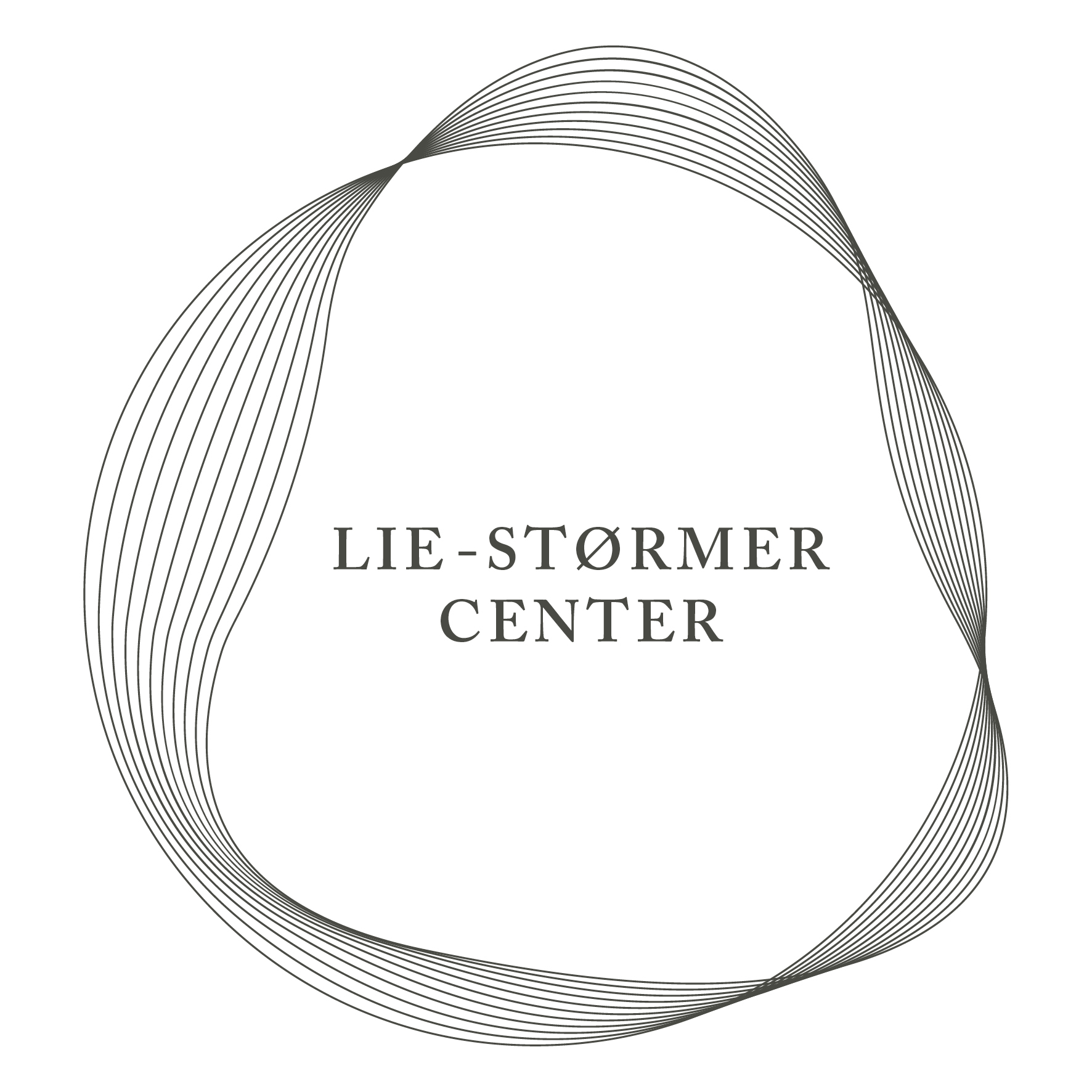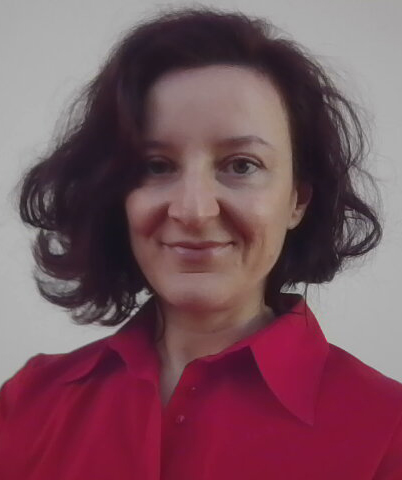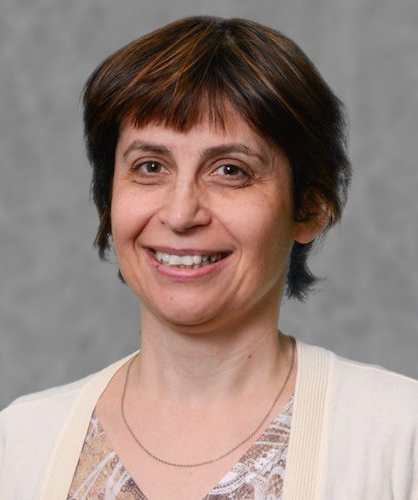# Tutorial Speakers

## Titles and Abstracts

Paola Boito
Università di Pisa, Italy### Matrix Structures and Matrix Functions

Abstract:Structured matrices play a relevant role in symbolic and numerical computations. In the literature and in applications we encounter several types of structure, which are typically related to the properties of the problems they stem from: banded structure is often associated with locality of functions or operators, Toeplitz structure arises from shift invariance properties, off-diagonal low-rank structure appears in inverses of banded matrices.

A common trait of most matrix structures is the availability of fast algorithms that perform fundamental operations, such as matrix-vector or matrix-matrix multiplication, solution of linear systems, or eigenvalue computation. For problems of large size, such algorithms are extremely useful both from a symbolic and a numeric point of view, although of course in a numerical setting one needs to pay attention to possible stability issues.

The tutorial will start with a brief overview of matrix structures and of their properties: we are especially interested here in rank structures and in certain forms of sparsity. We will then focus on selected topics concerning the analysis and computation of functions of structured matrices. Functions of matrices have a wide range of applications, for which we will give several examples, from the solution of differential equations to network analysis. Think for instance of the matrix exponential \$exp(A)\$: it appears in the solution of a multidimensional Cauchy problem with coefficient matrix \$A\$, but it also has a combinatorial meaning when \$A\$ is the adjacency matrix of a graph. If the quantity of interest is the action of a matrix function on a vector, moreover, one can often bypass the explicit construction of the matrix function itself and devise a more efficient approach.

Most methods for the computation of matrix functions are ultimately based on polynomial or rational approximation, which are either applied in explicit form, or through iterative methods such as Lanczos or Arnoldi, often in combination with suitable pre- and post-processing steps. If matrix \$A\$ is structured, it is natural to ask how to tailor such methods to the specific structure of \$A\$. We will present a few possible answers to this question and demonstrate their advantages through several practical examples.

Irina Kogan
North Carolina State University, USA### Invariants: Computation and Applications

Abstract: Invariants withstand transformations and, therefore, represent the essence of objects or phenomena. In mathematics, transformations often constitute a group action. Since the 19th century, studying the structure of various types of invariants and designing methods and algorithms to compute them remains an active area of ongoing research with an abundance of applications. In this incredibly vast topic, we focus on two particular themes displaying a fruitful interplay between the differential and algebraic invariant theories. First, we show how an algebraic adaptation of the moving frame method from differential geometry leads to a practical algorithm for computing a generating set of rational invariants. Then we discuss the notion of differential invariant signature, its role in solving equivalence problems in geometry and algebra, and some successes and challenges in designing algorithms based on this notion. Our goal is to convey the core ideas in an accessible and practical manner, often through examples, forgoing full generality, while providing references to more comprehensive expositions.

Laura Kovács
TU Wien, Austria### Algebra-Based Loop Analysis

Abstract: Automating loop analysis, and in particular synthesizing loop invariant, is a central challenge in the computer-aided verification of programs with loops, with applications in compiler optimization, probabilistic programming and IT security. While this challenge is in general undecidable, several techniques have emerged to automatically summarize the functional behaviour of software loops, thus providing inductive loop invariants that may prevent programmers from introducing errors while making changes in their code. In this tutorial, we show that novel combinations of methods from computer algebra, algorithmic combinatorics and static loop analysis provide powerful workhorses to derive (all) polynomial loop invariants, synthesize affine loops from invariants, and infer quantitative properties over the value distributions of probabilistic loop variables.

The key ingredients of our work root in (i) static analysis, to model loops as algebraic recurrence equations over the loop counter and to impose structural constraints over loops in order to guarantee that the resulting recurrences equations can be reduced to linear recurrences with constant coefficients; (ii) in symbolic summation and polynomial algebra, to solve recurrences and eliminate loop counters/recurrence variables by applying Groebner basis computation over closed-forms; (iii) in applied statistics, to compute quantitative loop invariants over higher-order statistical moments of polynomial probabilistic loops, and (iv) in automated reasoning, to simplify loop constraints and to use invariants towards ensuring functional correctness of loops.